# Introduction to zenplots

#### 2023-08-25

library(zenplots)

# All pairs

A zenplot can show the same information as a pairs plot but with two important display differences.

First, the matrix organization of the pairs layout is replaced by the “zig-zag” layout of zenplot. Second, the number of plots produced is about half that of a pairs plot allowing each plot in a zenplot to be given more visual space.

## Producing all pairs with PairViz

A convenient function to produce all pairs can be found in the PairViz package found on cran and installed in R via install.packages("PairViz").

library(PairViz)

We will illustrate this functionality and the difference between a pairs plot and a zenplot by first considering a small dataset on earthquakes having only a few variates. The difference between the two plots becomes much more important for data having larger numbers of variates – we illustrate the difference again using German data on voting patterns in two elections.

### Example: Ground acceleration of earthquakes

The built-in R data set called attenu contains measurements to estimate the attenuating effect of distance on the ground acceleration of earthquakes in California.

There are 5 different variates used to describe the peak acceleration of 23 California earthquakes measured at different observation stations. The data set contains 182 different peak acceleration measurements and has some missing data. The first few cases of the data set look like

head(attenu)
##   event mag station dist accel
## 1     1 7.0     117   12 0.359
## 2     2 7.4    1083  148 0.014
## 3     2 7.4    1095   42 0.196
## 4     2 7.4     283   85 0.135
## 5     2 7.4     135  107 0.062
## 6     2 7.4     475  109 0.054

Its variates are

##  "event"   "mag"     "station" "dist"    "accel"

and we are interested in all pairs of these variates.

To get these, first imagine a graph having as its nodes the variates of the data. An edge of this graph connects two nodes and hence represents a pair of variates. If interest lies in all pairs of varates, then the graph is a complete graph – it will have an edge between every pair of nodes. An ordering of variate pairs corresponds to any path on the graph. To have an ordering of all pairs of variates, the path must visit all edges and is called an Eulerian, or Euler path. Such a path always exists for complete graphs on an odd number of nodes; when the number of nodes is even, extra edges must be added to the graph before an Eulerian can exist.

For a complete graph with n nodes, the function eseq (for Euler sequence) function from the PairViz package returns an order in which the nodes (numbered 1 to n) can be visited to produce an Euler path. It works as follows.

## Since attenu has 5 variates, the complete graph has n=5 nodes
## and an Euler sequence is given as
eseq(5)
##   1 2 3 1 4 2 5 3 4 5 1

In terms of the variate names of attenu, this is:

names(attenu)[eseq(5)]
##   "event"   "mag"     "station" "event"   "dist"    "mag"     "accel"
##   "station" "dist"    "accel"   "event"

As can be seen in the corresponding complete graph below,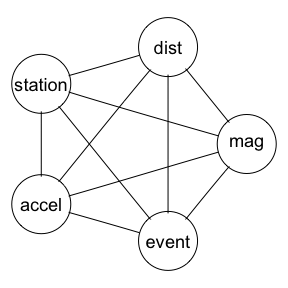this sequence traces an Eulerian path on the complete graph and so presents every variate next to every other variate somewhere in the order.

#### Euler sequences via zenpath

This functionality (and more) from PairViz has been bundled together in the zenplots package as a single function zenpath. For example,

zenpath(5)
##   5 1 2 3 1 4 2 5 3 4 5

This sequence, while still Eulerian, is slightly different than that returned by eseq(5). The sequence is chosen so that all pairs involving the first index appear earliest in the sequence, then all pairs involving the second index, and so on. We call this a “front loaded” sequence and identify it with the zenpath argument method = "front.loaded". Other possibilities are method = "back.loaded" and method = "balanced" giving the following sequences:

## Back loading ensures all pairs appear latest (back) for
## high values of the indices.
zenpath(5, method = "back.loaded")
##   1 2 3 1 4 2 5 3 4 5 1
## Frot loading ensures all pairs appear earliest (front) for
## low values of the indices.
zenpath(5, method = "front.loaded")
##   5 1 2 3 1 4 2 5 3 4 5
## Balanced loading ensures all pairs appear in groups of all
## indices (Hamiltonian paths -> a Hamiltonian decomposition of the Eulerian)
zenpath(5, method = "balanced")
##   1 2 3 5 4 1 3 4 2 5 1

The differences are easier to see when there are more nodes. Below, we show the index ordering (top to bottom) for each of these three methods when the graph has 15 nodes, here labelled”a” to “o” (to make plotting easier).

Starting from the bottom (the back of the sequence), “back loading” has the last index, “o”, complete its pairing with every other index before “n” completes all of its pairings. All of “n”’s pairings complete before those of “m”, all of “m”’s before “l”, and so on until the last pairing of “a” and “b” are completed. Note that the last indices still appear at the end of the sequence (since the sequence begins at the top of the display and moves down). The term “back loading” is used here in a double sense - the later (back) indices have their pairings appear as closely together as possible towards the back of the returned sequence. A simple reversal, that is rev(zenpath(15, method = "back.loaded")), would have them appear at the beginning of the sequence. In this case the “back loading” would only be in one sense, namely that the later indexed (back) nodes appear first in the reversed sequence.

Analogously, “front loading” has the first (front) indices appear at the front of the sequence with their pairings appear as closely together as possible.

The “balanced” case ensures that all indices appear in each block of pairings. In the figure there are 7 blocks.

All three sequences are Eulerian, meaning all pairs appear somewhere in each sequence.

#### Pairs plots versus zenplots

Eulerian sequences can now be used to compare a pairs plot with a zenplot when all pairs of variates are to be displayed.

First a pairs plot:

## We remove the space between plots and suppress the axes
## so as to give maximal space to the individual scatterplots.
## We also choose a different plotting character and reduce
## its size to better distinguish points.
pairs(attenu, oma=rep(0,4), gap=0, xaxt="n", yaxt="n")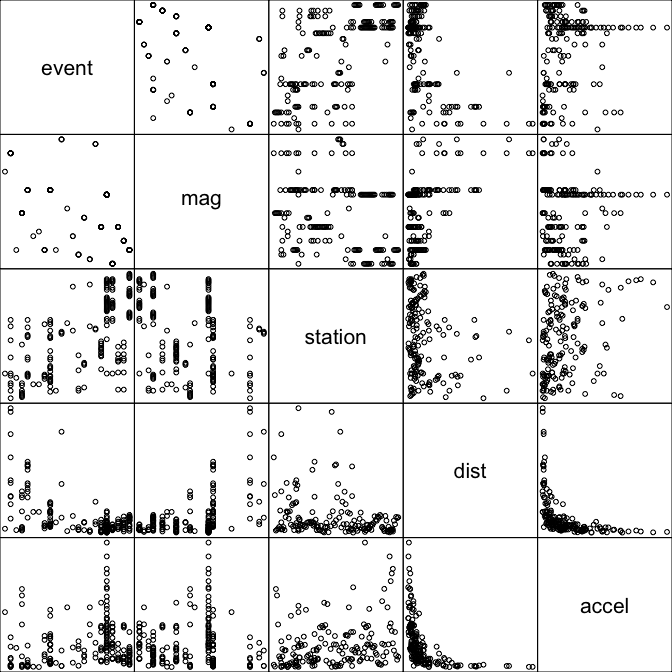We now effect a display of all pairs using zenplot.

## Plotting character and size are chosen to match that
## of the pairs plot.
## zenpath ensures that all pairs of variates appear
## in the zenplot.
## The last argument, n2dcol, is chosen so that the zenplot
## has the same number of plots across the page as does the
## pairs plot.
zenplot(attenu[, zenpath(ncol(attenu))], n2dcol=4)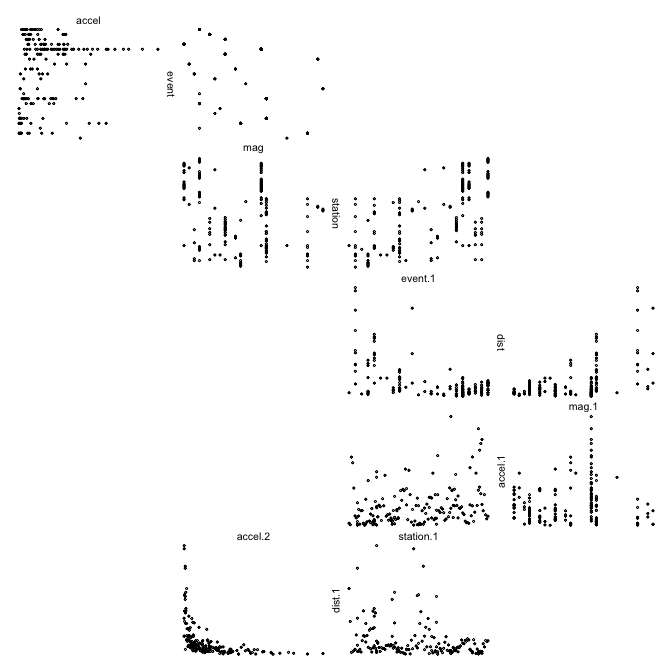Each display shows scatterplot of all choose(5,2) = 10 pairs of variates for this data. Each display occupies the same total area.

With pairs each plot is displayed twice and arranged in a symmetric matrix layout with the variate labels appearing along the diagonal. This makes for easy look-up but uses a lot of space.

With zenplot, each plot appears only once with its coordinate defining variates appearing as labels on horizontal (top or bottom) and vertical (left or right) axis positions. The layout follows the order of the variates in which the variates appear in the call to zenplot beginning in the top left corner of the display and then zig-zagging from top left to bottom right; when the rightmost boundary or the display is reached, the direction is reversed horizontally and the zigzag moves from top right to bottom left. The following display illustrates the pattern (had by simply calling zenplot):

## Call zenplot exactly as before, except that each scatterplot is replaced
## by an arrow that shows the direction of the layout.
zenplot(attenu[, zenpath(ncol(attenu))], plot2d="arrow", n2dcol=4)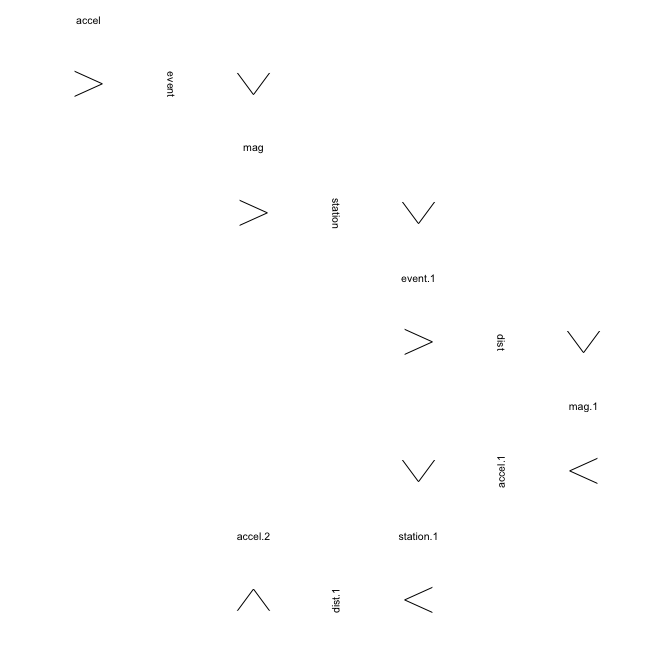The zig zag pattern of plots appears as follows.

• The top left plot (of either zenplot display) has horizontal variate event and vertical variate mag.
• To its right is a plot sharing the same vertical variate mag but now with horizontal variate station. Note that the variate station has some missing values and this is recorded on its label as station (some NA).
• Below this a plot appears with the same horizontal variate station but now with vertical variate event. Since this is the first repeat appearance of event it appears with a suffix as event.1.
• To its right is a plot with shared vertical variate event and new horizontal variate dist.
• Below this is a plot having shared horizontal variate dist and as vertical variate the first repeat of the variate mag.
• To its right is a plot having shared vertical variate mag and new horizontal variate accel.
• The right edge of the display is reached and the zigzag changes horizontal direction repeating the pattern until either the left edge is reached (whereupon the horizontal direction is reversed) or the variates are exhausted.

Like the pairs plot, the zenplot lays its plots out on a two dimensional grid – the argument n2dcol=4 specifies the number of columns for the 2d plots (e.g. scatterplots). As shown, this can lead to a lot of unused space in the display.

The zenplot layout can be made more compact by different choices of the argument n2dcol (odd values provide a more compact layout). For example,

## The default n2dcol is used
zenplot(attenu[, zenpath(ncol(attenu))], n2dcol=5)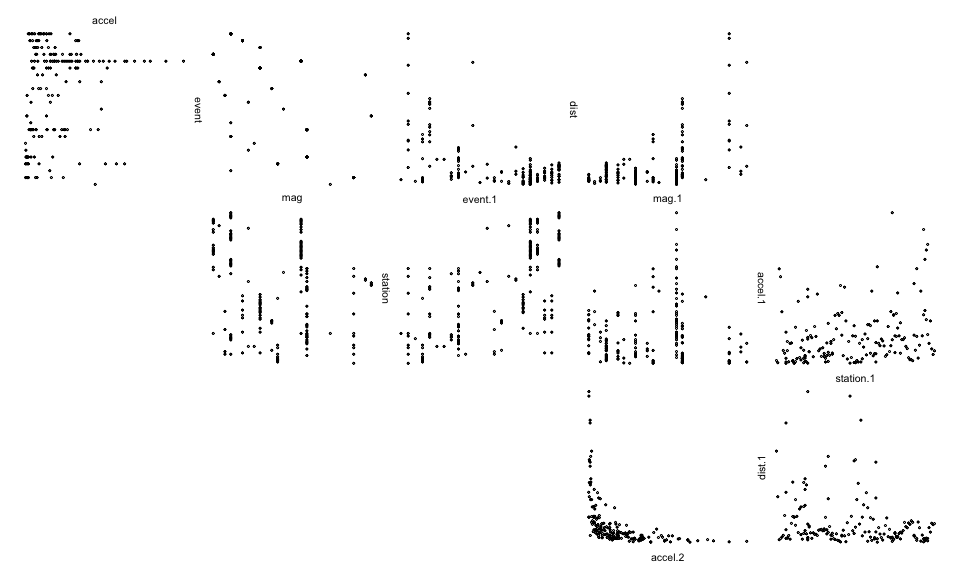By default, zenplot tries to determine a value for n2dcol that minimizes the space unused by its zigzag layout.

## The default n2dcol is used
zenplot(attenu[, zenpath(ncol(attenu))])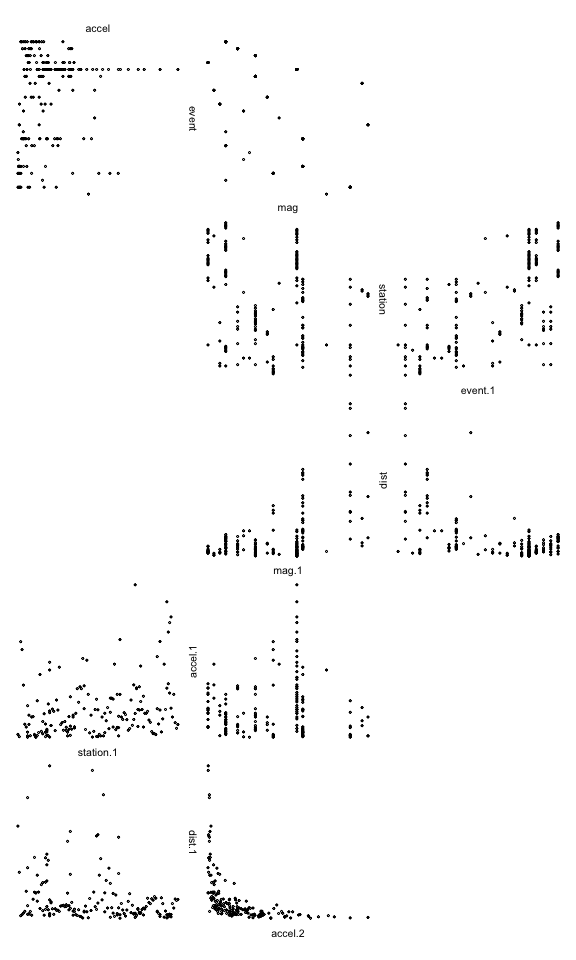with layout directions as

## The directions
zenplot(attenu[, zenpath(ncol(attenu))], plot2d="arrow")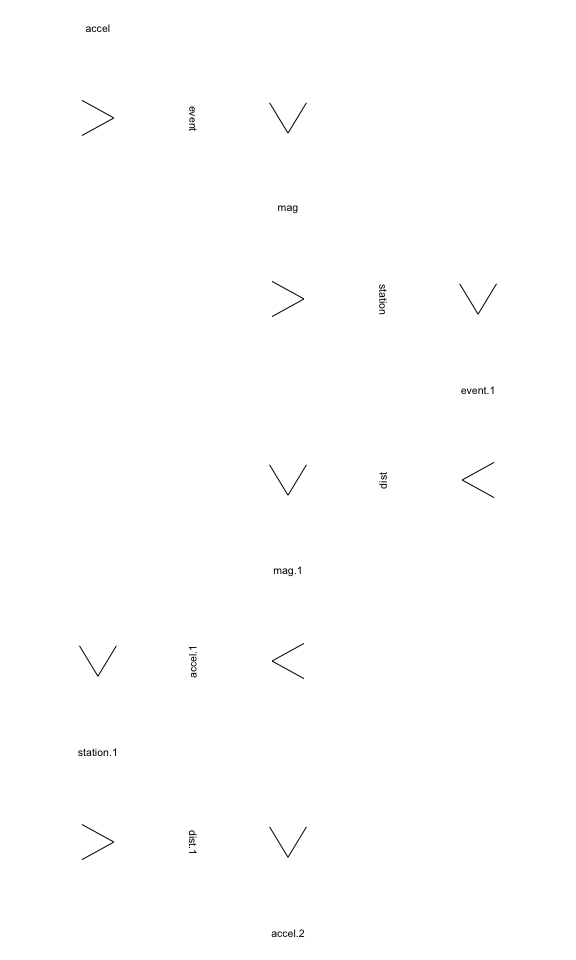As the direction arrows show, the default layout is to zigzag horizontally first as much as possible.

This is clearly a much more compact display. Again, axes are shared wherever a label appears between plots.

Unless explicitly specified, the value of n2dcol is determined by the aregument scaling which can either be a numerical value specifying the ratio of the height to the width of the zenplot layout or be a string describing a page whose ratio of height to width will be used. The possible strings are letter'' (the default),square’‘, A4'',golden’’ (for the golden ratio), or legal’’.

### Example: German election data

In the zenplots package the data set de_elect contains the district results of two German federal elections (2002 and 2005) as well as a number of socio-economic variates as well.

## Access the German election data from zenplots package
data(de_elect)

There are 299 districts and 68 variates yielding a possible choose(68,2) = 2278 different scatterplots.

This many scatterplots will overwhelm a pairs plot. In its most compact form, the pairs plot for the first 34 variates already occupies a fair bit of space:

## pairs(de_elect[,1:34], oma=rep(0,4), gap=0, pch=".", xaxt="n", yaxt="n")

(N.B. We do not execute any of these large plots simply to keep the storage needs of this vignette to a minimum. We do encourage the reader to execute the code however on their own.)

If you execute the above code you will see interesting point configurations including: some very strong positive correlations, some positive and negative correlations, non-linear relations, the existence of some outlying points, clustering, striation, etc.

Because this scatterplot matrix is for only half of the variates it shows choose(34,2) = 561 different scatterplots, each one twice. For a display of 1122 plots, only about one quarter of all 2278 pairwise variate scatterplots available in the data set appear in this display.

A second scatterplot matrix on the remaining 34 variates would also show only a quarter of the plots. The remaining half, $$34 \times 34 = 1156$$ plots, are missing from both plots.

In contrast, the zenplot shows all 2278 plots at once. In fact, because an Eulerian sequence requires a graph to be even (i.e. each node has an even number of edges), whenever the number of variates, $$p$$, is even zenpath(...) will repeat exactly $$p/2$$ pairs somewhere in the sequence it returns.

To produce the zenplot of all pairs of variates on the German election data we call zenplot(de_elect[,zenpath(68)], pch="."). (Again, we don’t produce it here so as to minimize the storage footprint of this vignette.)

## Try invoking the plot with the following
## zenplot(de_elect[,zenpath(68)], pch=".", n2dcol="square",col=adjustcolor("black",0.5))

In approximately the same visual space as the scatterplot matrix (showing only 561 unique plots), the zenplot has efficiently and compactly laid out all 2278 different plots plus r ncol(de_elect) duplicate plots. This efficient layout means that zenplot can facilitate visual search for interesting point configurations over much larger collections of variate pairs – in the case of the German election data, this all possible pairs of variates are presented simultaneously.

In contrast, all pairs loses most of the detail

## pairs(de_elect, oma=rep(0,4), gap=0, pch=".", xaxt="n", yaxt="n",col=adjustcolor("black",0.5))

# Groups of pairwise plots

Zenplots also accomodate a list of data sets whose pairwise contents are to be displayed. The need for this can arise quite naturally in many applications.

The German election data, for instance, contains socio-economic data whose variates naturally group together. For example, we might gather variates related to education into one group and those related to employment into another.

Education <- c("School.finishers",
"School.wo.2nd", "School.2nd",
"School.Real",  "School.UED")
Employment <- c("Employed", "FFF", "Industry",
"CTT", "OS" )

We could plot all pairs for these two groups in a single zenplot.

EducationData <- de_elect[, Education]
EmploymentData <- de_elect[, Employment]

## Plot all pairs within each group
zenplot(list(Educ= EducationData[, zenpath(ncol(EducationData))],
Empl= EmploymentData[, zenpath(ncol(EmploymentData))]))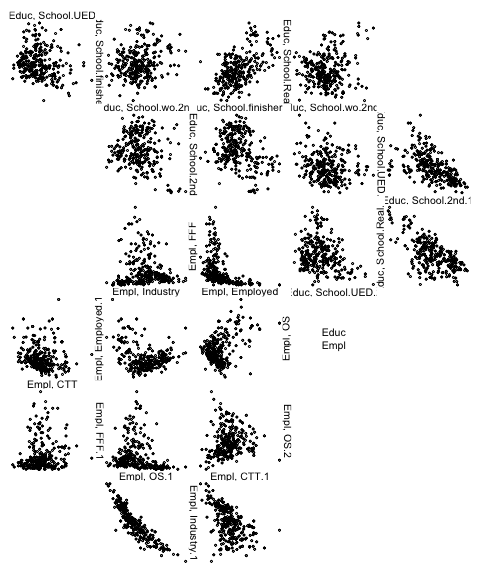All pairs of education variates are plotted first in zigzag order followed by a blank plot then continuing in the same zigzag pattern by plots all pairs of employment variates.

## All pairs by group

In addition to the Education and Employment groups above, a number of different groupings of variates having a shared context. For example, these might include the following:

## Grouping variates in the German election data
Regions <- c("District", "State", "Density")
PopDist <- c("Men", "Citizens", "Pop.18.25", "Pop.25.35",
"Pop.35.60", "Pop.g.60")
PopChange <- c("Births", "Deaths", "Move.in", "Move.out", "Increase")
Agriculture <- c("Farms", "Agriculture")
Mining <- c("Mining", "Mining.employees")
Apt <- c("Apt.new", "Apt")
Motorized <- c("Motorized")
Education <- c("School.finishers",
"School.wo.2nd", "School.2nd",
"School.Real",  "School.UED")
Unemployment <- c("Unemployment.03", "Unemployment.04")
Employment <- c("Employed", "FFF", "Industry", "CTT", "OS" )
Voting.05 <- c("Voters.05", "Votes.05", "Invalid.05", "Valid.05")
Voting.02 <- c("Voters.02", "Votes.02", "Invalid.02", "Valid.02")
Voting <- c(Voting.02, Voting.05)
PercentByParty.02 <- c("SPD.02", "CDU.CSU.02", "Gruene.02",
PercentByParty.05 <- c("SPD.05", "CDU.CSU.05", "Gruene.05",
PercentByParty <- c(PercentByParty.02, PercentByParty.05)

The groups can now be used to explore internal group relations for many different groups in the same plot. Here the following helper function comes in handy.

groups <- list(Regions=Regions, Pop=PopDist,
Change = PopChange, Agric=Agriculture,
Mining=Mining, Apt=Apt,  Cars=Motorized,
Educ=Education, Unemployed=Unemployment, Employed=Employment#,
# Vote02=Voting.02, Vote05=Voting.05,
# Perc02=PercentByParty.02, Perc05=PercentByParty.05
)

group_paths <- lapply(groups, FUN= function(g) g[zenpath(length(g), method = "front.loaded")] )
x <- groupData(de_elect, indices=group_paths)

zenplot(x, pch = ".", cex=0.7, col = "grey10")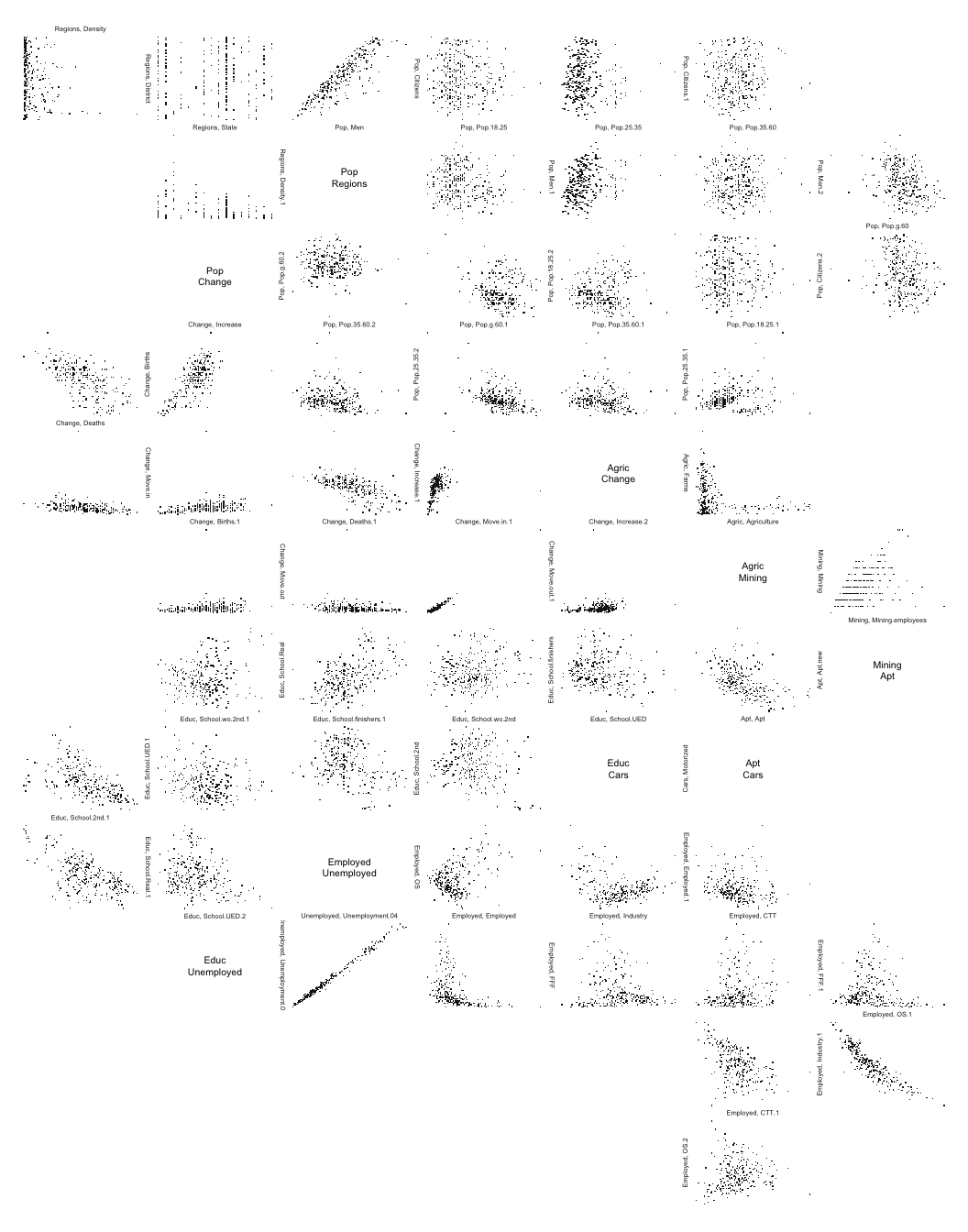All pairs within each group are presented following the zigzag pattern; each group is separated by an empty plot. The zenplot provides a quick overview of the pairwise relationships between variates within all groups.

The plot can be improved some by using shorter names for the variates. With a little work we can replace these within each group of x.

#
## Grouping variates in the German election data
RegionsShort <- c("ED", "State", "density")
PopDistShort <- c("men", "citizen", "18-25", "25-35", "35-60", "> 60")
PopChangeShort <- c("births", "deaths", "in", "out", "up")
AgricultureShort <- c("farms", "hectares")
MiningShort <- c("firms", "employees")
AptShort <- c("new", "all")
TransportationShort <- c("cars")
EducationShort <- c("finishers", "no.2nd", "2nd", "Real", "UED")
UnemploymentShort<- c("03", "04")
EmploymentShort <- c("employed", "FFF", "Industry", "CTT", "OS" )
Voting.05Short <- c("eligible", "votes", "invalid", "valid")
Voting.02Short <- c("eligible", "votes", "invalid", "valid")
PercentByParty.02Short <- c("SPD", "CDU.CSU", "Gruene", "FDP", "Linke", "rest")
PercentByParty.05Short <-  c("SPD", "CDU.CSU", "Gruene", "FDP", "Linke", "rest")

shortNames <- list(RegionsShort, PopDistShort, PopChangeShort, AgricultureShort,
MiningShort, AptShort, TransportationShort, EducationShort,
UnemploymentShort, EmploymentShort, Voting.05Short, Voting.02Short,
PercentByParty.05Short)
# Now replace the names in x by these.

nGroups <- length(x)

for (i in 1:nGroups) {
longNames <- colnames(x[[i]])
newNames <- shortNames[[i]]
oldNames <- groups[[i]]
#print(longNames)
#print(newNames)
for (j in 1:length(longNames)) {
for (k in 1:length(newNames)) {
if (grepl(oldNames[k], longNames[j])) {
longNames[longNames == longNames[j]] <- newNames[k]
}
}
}
colnames(x[[i]]) <- longNames
}

zenplot(x, pch = ".", cex=0.75)